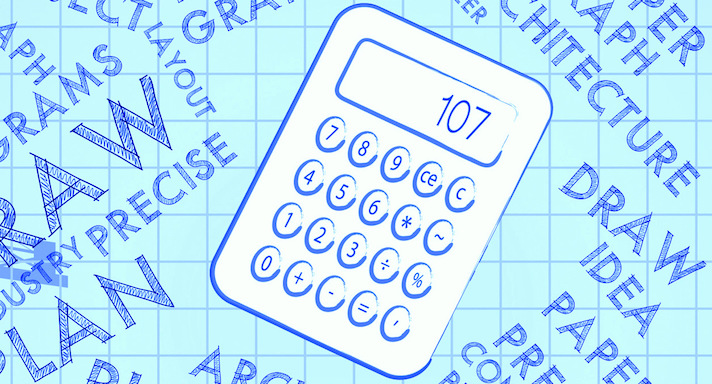# High School Math II-SE### Course Overview

Acellus High School Math II-SE provides Special Ed students with a basic mathematical foundation. Developing multiplication and division skills is a major focus in this course. Among the other topics covered in this course include fractions and an introduction to decimals. Acellus High School Math II-SE is taught by Acellus Instructors including Latricia Harper.This course was developed by the International Academy of Science. Learn More

### Scope and Sequence

Unit 1 In this unit students learn about the ones period, the thousands period, and the millions period. They explore comparing, the expanded form and the word form for the thousands period and the millions period. They study ordering numbers, and practice comparing numbers in story problems. Unit 2 In this unit students explore roman numerals, addition and subtraction, and fact families for addition and subtraction. They learn about parentheses with addition, properties of addition, and three-digit addition with regrouping. They discuss coin collecting, and explore four-digit addition with regrouping, as well as addition with different place values, choosing an appropriate method for addition, and three- and four-digit subtraction with regrouping. They continue with four-digit subtraction with zeros, subtraction with different place values, and choosing an appropriate method for addition and subtraction. They also practice addition and subtraction story problems. Unit 3 In this unit students focus on rounding, mental sums and differences, and estimating.  They study rounding in various periods, up to ten thousands.  They explore using rounding in estimating, over- and under-estimating, and compatible numbers, and they learn to determine whether they need an exact answer or an estimate. Unit 4 In this unit students gain understanding of multiplication and division. They study fact families, and multiplying and dividing by the numbers zero through ten, as well as 11, 15, and 12. They practice math fact drills and multiplication and division story problems, and explore properties of multiplication. They learn about expressions and equations, equations with variables, order of operations, and inequalities, and practice choosing an operation in story problems. Unit 5 In this unit students focus on time, temperature, and negative numbers. They learn to tell time to the nearest minute and second, and explore different ways to say the same time. They practice rounding with time and learn the names of months. They study elapsed time and units of time. They investigate using a thermometer, as well as using negative numbers, and learn to compare units of time, different temperatures, and negative numbers. Unit 6 In this unit students' emphasis is on multiplication using one, two, three, and four digits. They practice mental math, estimating, rounding, and compatible numbers. They explore multiplication with and without regrouping, as well as multipying by ten, story problems, and selecting an appropriate multiplication method. Unit 7 In this unit students focus on statistics. They investigate data tables, frequency tables, and venn diagrams, as well as line plots, bar and double-bar graphs, pictographs, line graphs, circle graphs, and coordinate grids. In addition, they study range, mean, median, and mode. Following this unit students are presented with the Mid-Term Review and Exam. Unit 8 In this unit students discover division. They discuss mental math, missing numbers, estimating, rounding, compatible numbers, and remainders, all as they pertain to division. They learn to divide using one, two, and three digits, and study division story problems with remainders. Unit 9 In this unit students continue their exploration of division. They learn about dividing with zeros, using multiplication to check division, and choosing an appropriate method. They study mental math and estimating, and practice two-, three-, and four-digit division problems, as well as adjusting quotients, story problems with a map, and story problems for choosing the operation. Unit 10 In this unit students turn to fractions. They learn about number lines, equivalent fractions and models, reducing fractions to simplest form, comparing like and unlike fractions, and ordering fractions. They study mixed numbers and improper fractions, as well as adding, subtracting, and multiplying with fractions, mixed numbers, and whole numbers. Unit 11 In this unit students explore decimals. They learn about decimals on the number line, and how decimals, fractions, and mixed numbers are related. They study expanded forms and word forms of decimals, as well as comparing, ordering, estimating, and rounding decimals. They investigate adding and subtracting decimals, and practice story problems. Unit 12 In this unit students study money. They discuss counting, rounding and estimating with money, as well as adding, subtracting, multiplying and dividing with money. They gain understanding of fractions and decimals as they relate to money, and practice money story problems. Unit 13 In this unit students explore geometry. They study points, lines, rays, angles, polygons, triangles, quadrilaterals, circles, and solid figures. They learn about terms, labeling, measuring, classifying, line relationships, similar and congruent figures, symmetry, faces, edges, and vertices. Unit 14 In this unit students learn about measurement of length, weight, and mass. They study customary and metric units, comparing and changing units, and measurement story problems. Unit 15 In this unit students study measurement of capacity, perimeter, area of squares and rectangles, and volume. They explore customary and metric units, and changing customary and metric units. Unit 16 In this unit students explore probability, divisibility, factors and multiples, prime and composite numbers, and number patterns. Students also study tree diagrams, line plots with fractions, and story problems about working backward. Following this unit, students are presented with the Final Review and Exam.